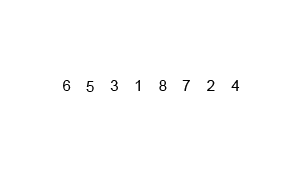# 35. Merge Sort¶

The merge sort algorithm is a recursive algorithm based on the divide and conquer technique.

It was developed in 1945 by John Von Neumann and adapts very well to the ordering of large amounts of sequential data because it is very fast and works easily with data that can only be accessed one at a time.

## Algorithm Description¶

The merge sort algorithm is based on recursively dividing the list of data to be sorted into two sublists each half the size of the original, in order to sort them more easily.

When the list is of size 0 or 1 it will already be ordered.

Once the two sublists are sorted, an algorithm is applied that merges the two sorted sublists into a single sorted list of double size.

In this way, sublists of increasing size are mixed until the entire original list is ordered.Merge sort algorithm.

Swfung8, CC BY-SA 3.0 Unported, via Wikimedia Commons.

## Management program¶

Below is the code for sorting elements of a list according to the merge algorithm:

```def ordenar(lista, inicio=0, final=None):
if final == None:
final = len(lista) - 1

if inicio == final:
return

medio = (inicio +  final + 1) // 2
ordenar(lista, inicio, medio - 1)
ordenar(lista, medio, final)
mezclar(lista, inicio, medio, final)

def mezclar(lista, inicio, medio, final):
while (inicio < medio and inicio < final and medio <= final):
if lista[medio] < lista[inicio]:
temp = lista[medio]
i = medio
while (i > inicio):
lista[i] = lista[i - 1]
i = i - 1
lista[inicio] = temp
medio = medio + 1
inicio = inicio + 1

lista = [6, 3, 9, 5, 7, 1, 2, 4, 10, 8]
ordenar(lista)
print(lista)
```

Exit:

```[1, 2, 3, 4, 5, 6, 7, 8, 9, 10]
```